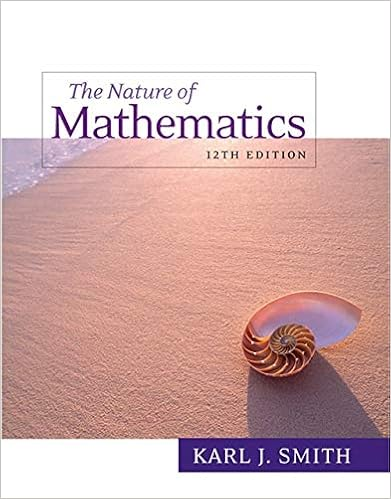• Homework Help
• 4
• 0% (2) 0 out of 2 people found this document helpful

This preview shows page 1 - 2 out of 4 pages.

##### We have textbook solutions for you!
The document you are viewing contains questions related to this textbook.The document you are viewing contains questions related to this textbook.
Chapter 13 / Exercise 3
Nature of Mathematics
SmithExpert Verified
STAT 225 Spring 2014 ---Graded Homework 1 -------DUE in class on Wednesday, Feb. 5, 2014. Your instructor will give you further information on the format of the homework. SHOW WORK!!!!! 1.A person may be able to snap his/her fingers. Likewise, a person may be able to whistle. Let A be the event that a person can snap his/her fingers. Let B be the event that a person can whistle. We have P(A) = 0.62 and P(B) = 0.71 a)What is the probability of the largest intersection of A and B? b)It is known that P(Ac ∩ Bc) = 0.15. What does Ac ∩ Bcrepresent? (Write this out in words). What is P(A ∩ B)using P(Ac ∩ Bc) = 0.15? 2.A bike lock uses a three digit code, utilizing the digits 0 through 9. a)How many possible codes are there to open the lock? b)If you may not repeat a digit, how many possible codes are there? c)Assume that the first two digits must be one 3 and one 4 (in either order)list the sample space of codes. d)Using the sample space in 2c)----what is the probability that a code has no digits repeated? e)Using the sample space in 2c)----what is the probability that a code ends in a digit greater than 6? 3.JJ’s Video Game store sells used games. For Pokémon fans, the store has used copies of Pokémon Pearl, Diamond and Ruby for sale. 22% of the Pokémon games sold are Pearl, 36% are Diamond and the rest are Ruby. Annie knows that counterfeit (i.e. fake) games are commonly sold used. She has done some research on this and has found that a used Diamond game will be fake with a probability of 0.18, a used Pearl game will be fake with a probability of 0.1 and a used Ruby game will be fake with a probability of 0.26. Find the probability that a customer buying a used Pokémon game at this store will purchase a counterfeit Pokémon game. 4.You have a bag full of apples.
##### We have textbook solutions for you!
The document you are viewing contains questions related to this textbook.The document you are viewing contains questions related to this textbook.
Chapter 13 / Exercise 3
Nature of Mathematics
SmithExpert Verified
•••